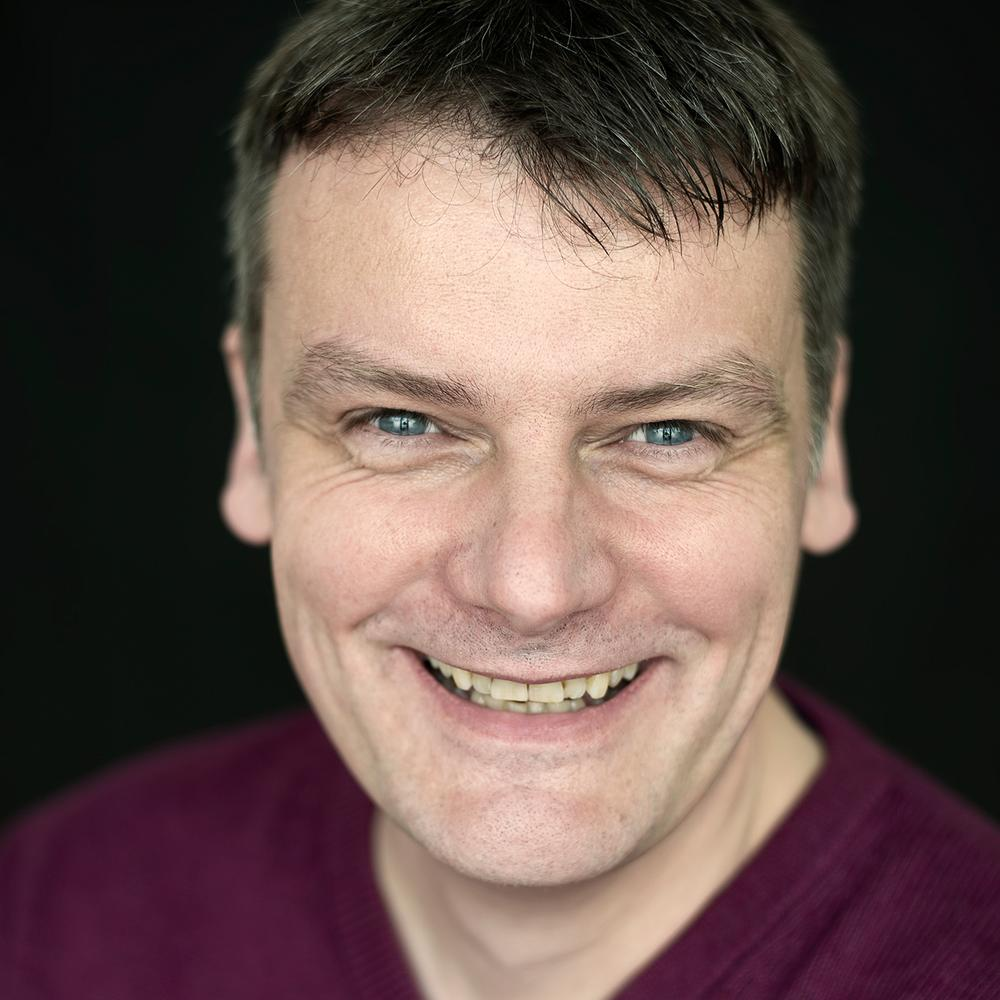# Standard Form

### Standard Form – What is it?

Standard Form is a way to write really large or really small numbers. It is a way to write these numbers in a format that everyone agrees to.

### What’s in a name?

Although the idea is to write the numbers into a set format, the name of the format is not always agreed on. You may hear terms such as Standard Index Form, Scientific Notation, Standard Form and SIF. However, they are all the same. The one to watch is Engineering notation which is similar but does not follow the same rules.

INTEGRATION IN MATHS: THE PADDINGTON BEAR APPROACH

### Where did they come from?

A famous mathematician and Greek philosopher called Archimedes was thought to have used them initially. He used them to help him try to calculate the size of the universe.

### Why do we need it?

As we get more and more advanced we are often dealing with very large numbers. This is not just bank balances! Science is also a key area where large numbers are used. For instance, when talking about the distance between planets or even galaxies we often need to use large numbers.

Equally, as science explores the smaller world such as atoms, particles and quantum physics, then we are often using ridiculously small measurements and we need a way of writing these numbers down.

If we just write these large or small numbers normally (often referred to as ordinary form) then it is often difficult to count or quickly see the number of 0’s. If we miscount or accidentally miss one-off, then it could make a dramatic difference to any calculations we are trying to do. Just imagine if you were expecting £100 but were given £10, you might not be too happy. When numbers are 30 or 40 digits off then it is easy to make a mistake.

### What does a number in Standard Form look like?

A number is Standard Form has to follow a set of 3 rules which everyone uses.You can see that from this that the first part is a number between 1 and 10. This number can be 1 and go up to but not include 10. We sometimes write this as 1≤n<10. The number can be an integer (whole number) or a decimal.

##### Fun Fact – A little known fact is that this number has a special name. It is called the mantissa! Not a lot of people know that.

The second part is always the “x10” and this is always the same. This is because our place value system is based on 10 so by multiplying by 10 we are moving the digits one place left. For example, 8 becomes 80 and 1.23 becomes 12.3 .

The last part of the number is the power. This is sometimes called the index or exponent. This is placed on the “x10”. This has to be an integer but can be positive or negative. If it is positive, it means we are multiplying by 10 lots of times. So, for example, a power of 3 would multiply the number by 10 three times. If the power is 24, then we are multiplying by 10 24 times (which will make a very large number).

If the power is negative, then we are effectively dividing by 10 that number of times. For example, a power of -6 would suggest we are dividing by 10 six times.

So in the example above we are starting with the number 4.2 and multiplying it by 10 nine times. This means we are really doing

4.2 x 10 x 10 x 10 x 10 x 10 x 10 x 10 x 10 x10

or

4.2 x 1,000,000,000 which is 4,200,000,000

HOW TO FACTORISE AND SOLVE QUADRATIC EQUATIONS

### Skills needed for GCSE

For GCSEs, you need to be able to convert numbers to and from Standard Form. If you can multiply and divide numbers by 10 then you should be able to do this without a calculator.

You also need to be able to compare numbers in Standard Form. This is done by looking at the power. The bigger the power, the bigger the number. Remember that small numbers will have a negative power.

Complete calculations with Standard Form. You need to be able to complete simple calculations with Standard Form. This could be both with and without a calculator. Your calculator has a special button on it for Standard Form.

Want your child to succeed? Why not try one of our expert tutors. You can read why this helps here: Why Should I book a Maths Tutor

###A bit about Phil:

Phil offers tutoring up to secondary school aged students, up to GCSE, in Maths. Having taught GCSE maths for many years, he can help both Foundation Level and Higher-Level students improve their grades.

He is currently a teacher in a secondary school in Colchester and has been teaching maths for over 22 years. He is a fully qualified teacher with a degree (BSc) in Maths and Computer Science.

You can enquire about tutoring with Phil here## The Tutor Team Guarantee

We will never offer your child an unqualified student, someone without a police check, or anyone who isn’t experienced.
We only work with highly-qualified, experienced tutors.